1. Kindly refer the question below:

When a nucleus undergoes radioactive decay, the change in the number of particles in the universe is

2. The diagram shows four possible electron energy levels in the hydrogen atom.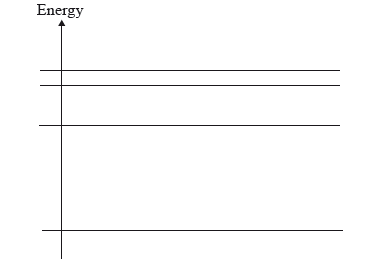The number of different frequencies in the emission spectrum of atomic hydrogen that arise from electron transitions between these levels is

3. All the energy levels in a simple model of an atom are shown.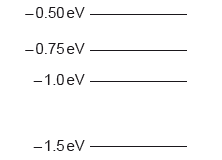The atom is excited so that an electron is promoted to the energy level. How many different frequencies will be observed in the emission spectrum?

4. A nucleus of the isotope potassium-40 decays to a nucleus of the isotope argon-40.

The reaction equation for this decay may be written as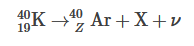Which of the following correctly identifies the proton number of argon-40 and the particle X?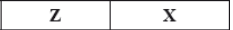5. Which of the following lists the particles emitted during radioactive decay in order of increasing ionizing power?
6. What is correct about the Higgs Boson?
7. Kindly refer the question below: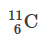undergoes decay. The products of this decay are the particle, X and Y. What are X and Y?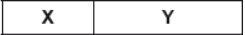8. Which of the following leads to a paradigm shift?
9. Which of the following would decrease the initial activity of a sample of plutonium?
10. Which of the following gives evidence to support the existence of atomic energy levels?
11. Which of the following is a correct list of particles upon which the strong nuclear force may act?
12. All isotopes of uranium must have the same
13. Identify the conservation law violated in the proposed reaction.

p+ + p+ → p+ + n0 + μ+

14. Patterns in graphs help scientists make predictions. What can be deduced from a graph of neutron number versus proton number for all stable nuclides?
15. A pure sample of nuclide A and a pure sample of nuclide B have the same activity at time t = 0. Nuclide A has a half-life of T, nuclide B has a half-life of 2T.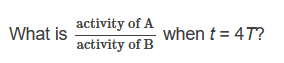16. Protons and neutrons are held together in the nucleus by the
17. Kindly refer the question below:

A radioactive isotope has an initial activity and a half-life of 1 day. The graph shows how the activity varies with time. time.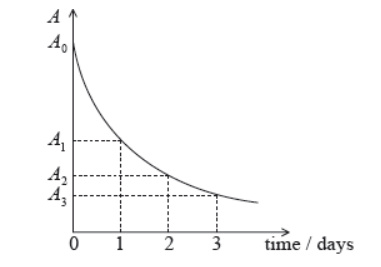The ratio A_0/A_2 is equal to which of the following?

18. A pure sample of a known element has a very long half-life. What measurement(s), together with the initial activity of the sample, must be made in order to measure the half-life of the element?
19. The de Broglie wavelength of an electron is equal to the wavelength of a photon that has energy E. What is the momentum of the electron?
20. A unit in which mass defect can be measured is
21. The nuclear reaction represented by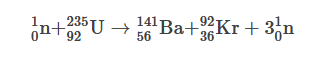is an example of

22. A proton decays to a neutron. The other products of the decay are a
23. Evidence for the existence of isotopes can come from analysis of
24. The half-life of a radioactive nuclide is 20s. What fraction of the original sample will have decayed in one minute?
25. The energies of alpha particles and of gamma-rays emitted in radioactive decay are discrete. This observation is evidence for
26. A radioactive nuclide decays to a stable daughter nuclide. Initially the sample consists entirely of atoms of the radioactive nuclide. What fraction of the sample consists of the daughter nuclide after four half-lives?
27. The lowest four energy levels of a particular atom are represented in the energy level diagram below.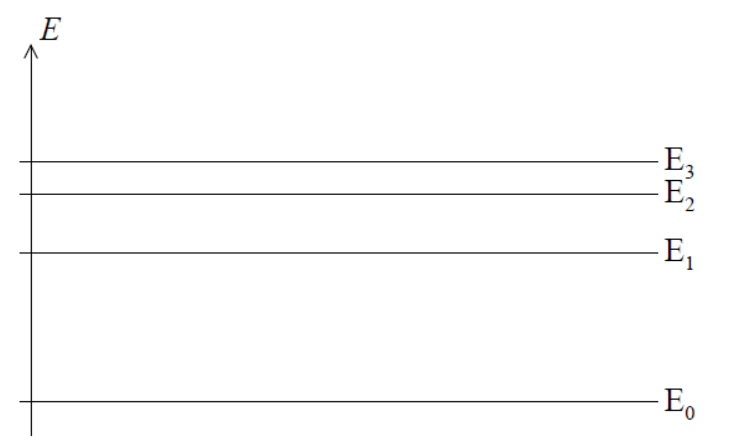Planck’s constant is h. What is the highest frequency in the atom’s emission spectrum that is associated with these levels?

28. The graph shows the variation with time t of the activity A of a radioactive sample. The energy released in each decay is E. The shaded area is equal to S.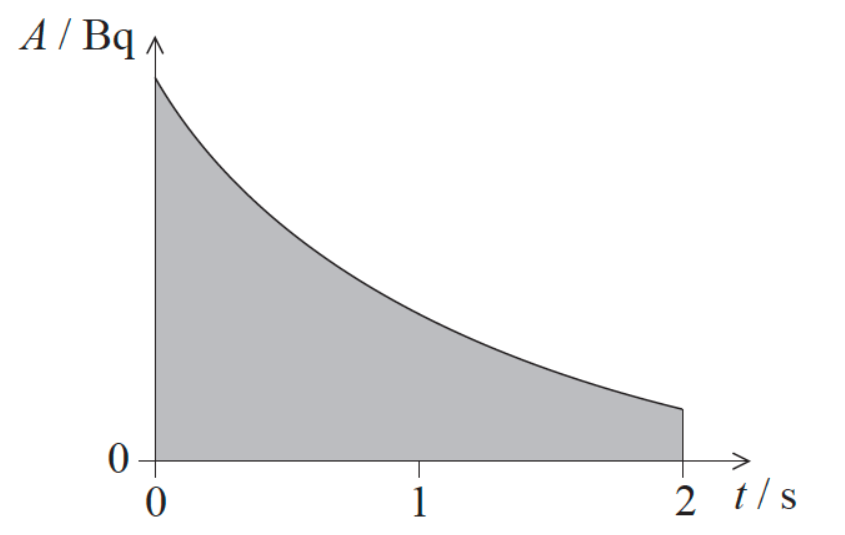What does the quantity S E represent?

29. The diagram shows three electron energy levels of an atom. Which transition results in the emission of a photon of the longest wavelength?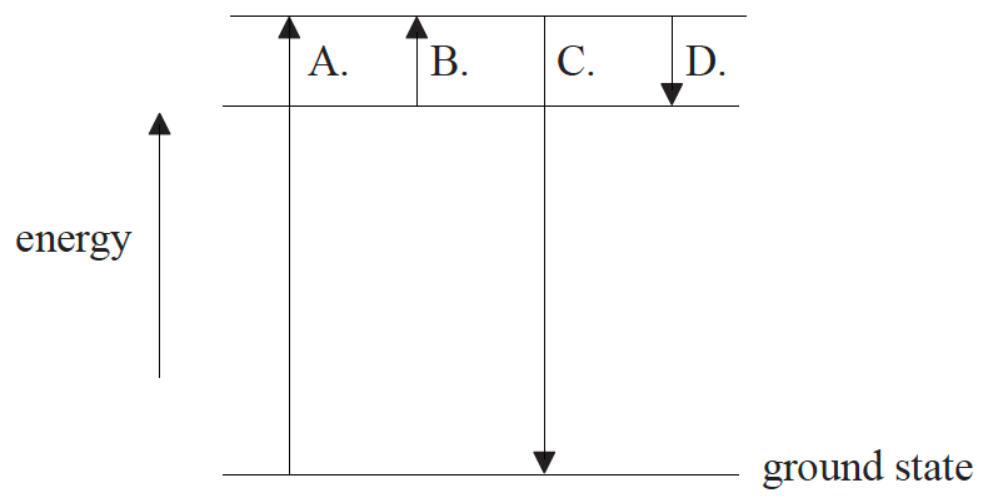30. When compared with beta particles and gamma-ray photons, alpha particles have the greatest
31. An alpha particle is directed head-on towards a nucleus of an isotope of iron. A second alpha particle, with the same energy as the first, is directed head-on towards a different isotope of iron.

Which of the following is a comparison of the distances of closest approach of the two alpha particles and the forces experienced by the alpha particles at the point of closest approach?32. In the nuclear reaction X + Y → Z + W, involving nuclides X, Y, Z and W, energy is released. Which is correct about the masses (M) and the binding energies (BE) of the nuclides?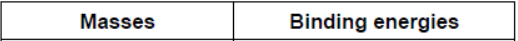33. What is the charge on an electron antineutrino and during what process is an electron antineutrino produced?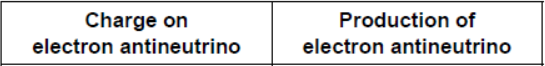34. The arrows below indicate transitions involving three energy levels of an atom. The wavelength of the photon emitted in each transition is indicated.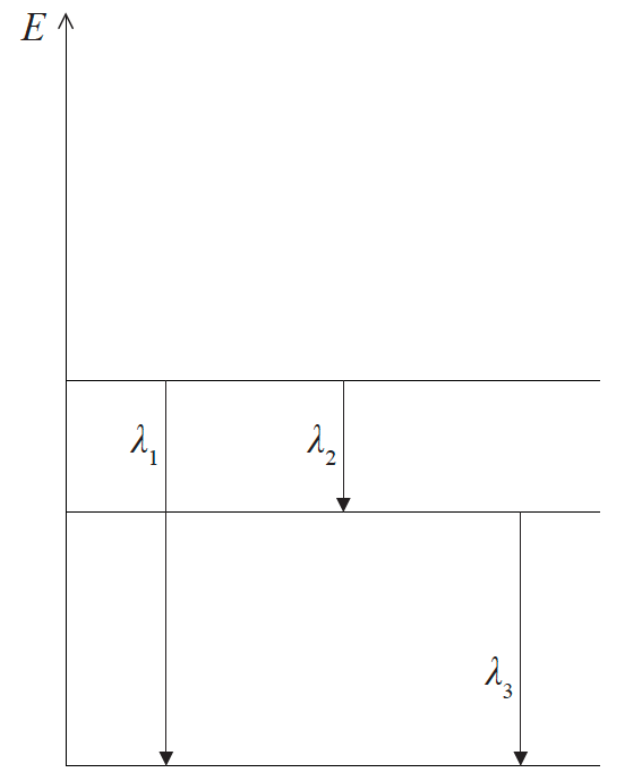Which of the following relationships between the wavelengths is correct?

35. A fission reaction for uranium is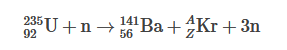where n is the neutron. Which of the following gives the value of the nucleon number A and proton number Z for the krypton (Kr)?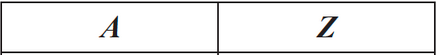36. The diagram below shows some of the energy levels available to an electron in a caesium atom.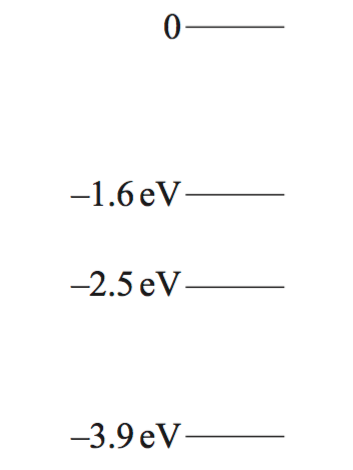Photons of energy 0.9eV pass through a sample of low pressure caesium vapour. Which of the following gives the energy transition of the electron when a photon is absorbed?

37. The nuclei in a sample of a radioactive isotope decay by emitting α and γ particles. Which of the following is correct for the energies of the α particles and for the energies of the γ particles?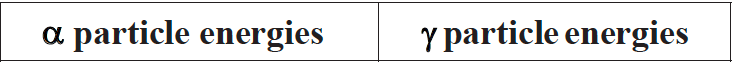38. The graph shows the relationship between binding energy per nucleon and nucleon number. In which region are nuclei most stable?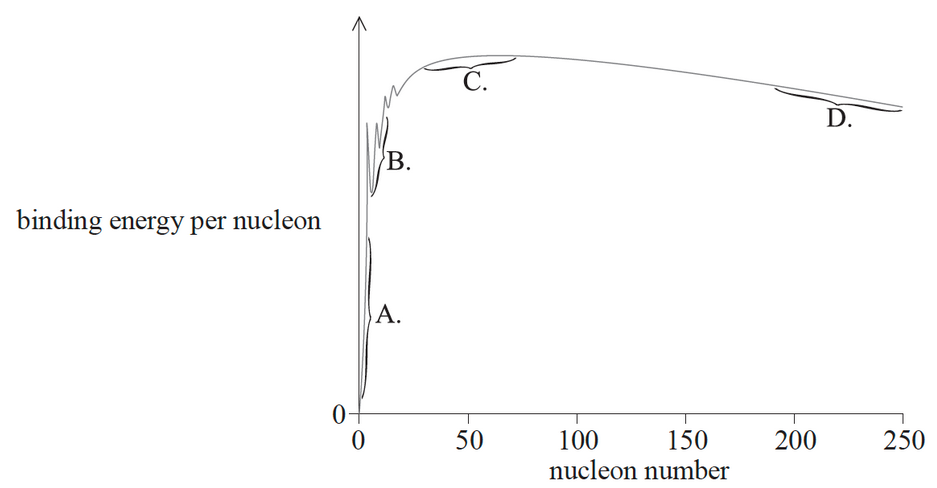39. The diagram shows the three lowest energy levels of an atom.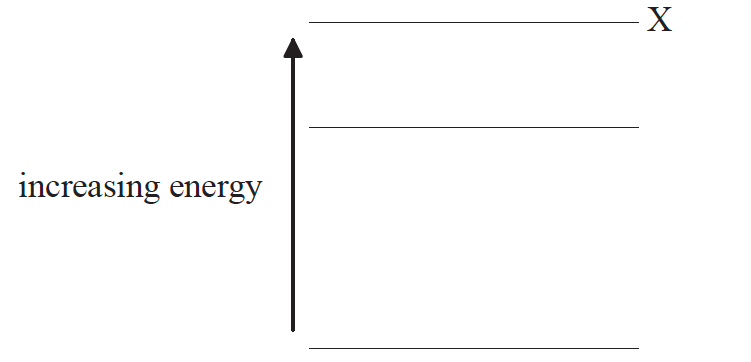Which diagram shows the emission line spectrum associated with electron transitions from energy level X?

40. Some of the energy levels for a hydrogen atom are shown in the diagram.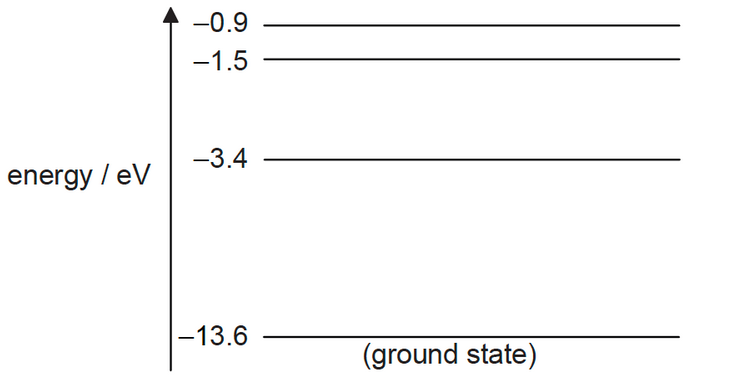The table shows four photons with their corresponding energies.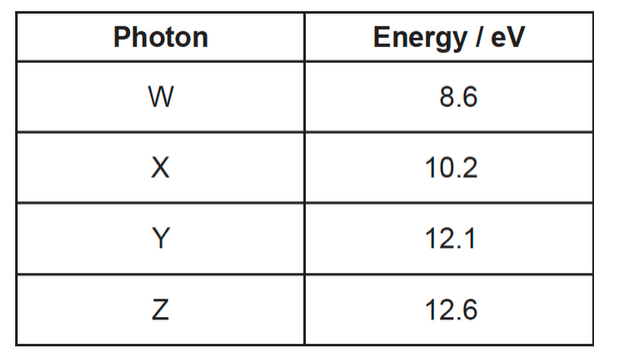Each photon is incident on a hydrogen atom in its ground state. Which photons could be absorbed by the atom?

41. The diagram shows four energy levels W, X, Y and Z of an atom.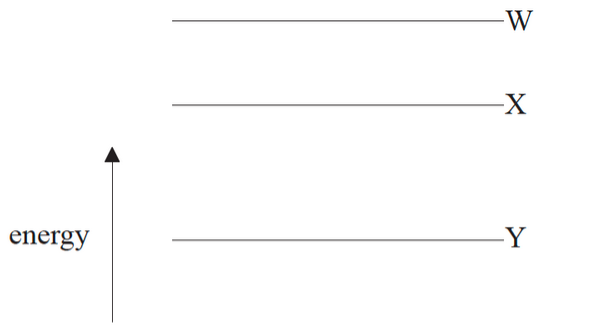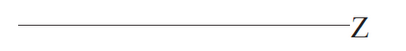Which electron transition will produce a photon of the longest wavelength and which transition will produce a photon with the highest frequency?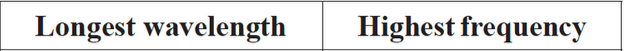42. In a fission reaction,

the total mass and the total binding energy before the reaction are Mi and Ei respectively, where the binding energy is defined as a positive quantity. After the reaction the total mass is Mf and the total binding energy is Ef . Which of the following correctly compares the total masses and the total binding energies?43. The diagram shows three energy levels of the hydrogen atom and some of the associated electron transitions between the levels.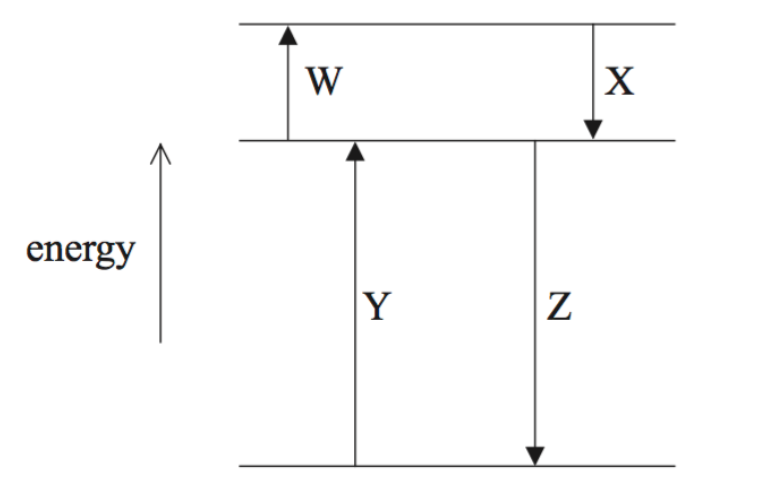Which labelled electron transition gives rise to the photon with the greatest wavelength and which gives rise to the photon with the smallest wavelength?